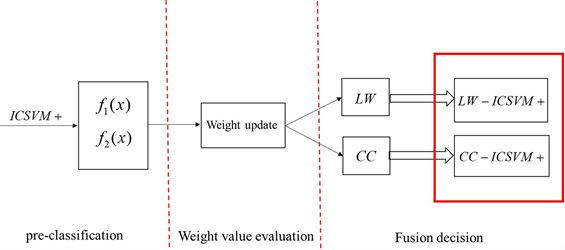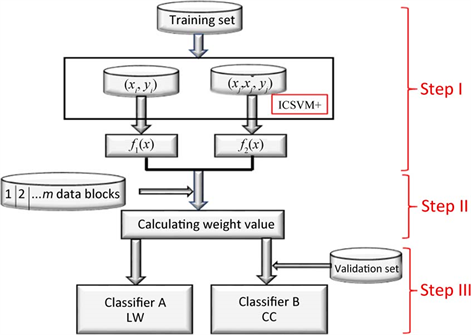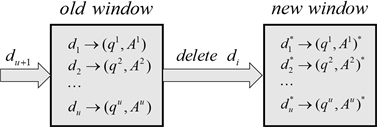﻿ 基于部分特权信息的支持向量机

# 基于部分特权信息的支持向量机Support Vector Machines Based on Incomplete Privileged Information

Abstract: The importance of privileged information is particularly prominent in many fields, especially in bio-medicine. It is because researchers have proven that privileged information can improve clas-sification accuracy. And the traditional approach usually uses SVM+ to solve the classification problem that contains privileged information. Unfortunately, in many cases the training data set contains only partial privileged information due to the high cost of privileged information collection. In the face of data with incomplete privilege information, the biggest challenge is how to use this partial privilege information to obtain the maximum hidden information of the data. Fortunately, a new fusion classification algorithm based on incomplete privileged information for Support Vector Machine (ICSVM+) that we proposed, can solve this problem well. In this paper, two ideas are proposed for the fusion classification algorithm, namely, cross-correction (CC) and linear weighting (LW). We call these two methods (CC) ICSVM+ and (LW) ICSVM+. Another important work is that a weight updating method based on window theory proposed by us can adapt well to different data sets. Through experimental comparison with SVM and SVM+, our ICSVM+ methods were able to effectively deal with the lack of privilege information.

1. 引言

Vapnik (2009)提出了基于SVM   的新学习范式，即学习使用特权信息(LUPI) ，被称为基于特权信息的支持向量机(SVM+)。训练阶段提供特权信息，但不适用于测试集。Vapnik对特权信息做了大量的研究，并且被运用在各个领域中     。实验结果表明，学习使用正确的特权信息有助于提高分类性能 。在某些情况下，特权信息很难收集而且昂贵，特别是在生物学和医学。因此，在实际数据中，有许多情况下只有部分训练样本具有特权信息 。由于SVM+不适合这种情况，我们必须构建一个适应具有部分特权信息的数据集的新模型。部分特权信息类似于通常已知的缺失数据集。对于缺少的数据集，我们现阶段有许多方法完成  。使用其他信息和特权信息的关联来完成缺失的特权信息也是一个可行的方法。这种情况更适合Pu J等人研究的医学数据 。然而，它需要获得特征和标签之间的关系，以补充特权信息的缺失数据，这可能在生物医学中具有更好的实验性能。但在其他领域，特征和标签之间的关系在特权信息中不能很好的体现。因此，这样来补充缺失的数据集可能无法获得稳定的实验结果。Figure 1. LW-ICSVM+ and CC-ICSVM+ implementation process. The red box is the two classification methods proposed in this paper. LW is a linear weighted fusion method and CC is a cross-correction fusion method

2. 相关工作

3. 算法介绍

3.1. 基于SVM的部分特权信息

SVM+实现了具有特权信息的数据分类问题，但没有解决部分特权信息的应用。本文提出的模型称为ICSVM+可以更好地解决问题。当只有一部分样本有可用特权信息，其他n个样本没有特权信息，给定一组三维特征样本：

$\left({x}_{1},{y}_{1}\right),\cdots ,\left({x}_{n},{y}_{n}\right),\left({x}_{n+1},{x}_{n\text{+1}}^{\text{*}},{y}_{n+1}\right),\cdots ,\left({x}_{l},{x}_{l}^{\text{*}},{y}_{l}\right),\text{\hspace{0.17em}}x\in X,{x}^{*}\in {X}^{*},y\in \left\{-1,1\right\}.$

$\begin{array}{l}\underset{\omega ,{\omega }^{\ast },b,{b}^{\ast }}{\mathrm{min}}\text{ }\text{ }\text{ }\text{ }\text{ }\text{ }\text{ }\text{ }\text{ }\text{ }\text{ }\frac{1}{2}\left[\left({w}_{1}\cdot {w}_{1}\right)+\left({w}_{2}\cdot {w}_{2}\right)+\gamma \left({w}^{\ast }\cdot {w}^{\ast }\right)\right]+C\underset{i=1}{\overset{n}{\sum }}{\xi }_{i}+{C}^{\ast }\underset{i=n+1}{\overset{l}{\sum }}\left[\left({w}^{\ast }\cdot {z}_{i}^{\ast }\right)+{b}^{\ast }\right]\\ \text{ }\text{ }\text{ }\text{ }\text{ }\text{ }\text{ }\text{ }\text{ }s.t.\text{ }\text{ }\text{ }\text{ }\text{ }\text{ }\text{ }\text{ }\text{ }\text{ }\text{ }\text{ }\text{ }\text{ }\text{ }\text{ }{y}_{i}\left[\left({w}_{1}\cdot {z}_{i}\right)+{b}_{1}\right]\ge 1-{\xi }_{i},i=1,\cdots ,n\\ \text{ }\text{ }\text{ }\text{ }\text{ }\text{ }\text{ }\text{ }\text{ }\text{ }\text{ }\text{ }\text{ }\text{ }\text{ }\text{ }\text{ }\text{ }\text{ }\text{ }\text{ }\text{ }\text{ }\text{ }\text{ }\text{ }\text{ }\text{ }\text{ }\text{ }\text{ }\text{ }\text{ }\text{ }\text{ }\text{ }{y}_{i}\left[\left({w}_{2}\cdot {z}_{i}\right)+{b}_{2}\right]\ge 1-\left[\left({w}^{\ast }\cdot {z}_{i}^{\ast }\right)+{b}^{\ast }\right],i=n+1,\cdots ,l\\ \text{ }\text{ }\text{ }\text{ }\text{ }\text{ }\text{ }\text{ }\text{ }\text{ }\text{ }\text{ }\text{ }\text{ }\text{ }\text{ }\text{ }\text{ }\text{ }\text{ }\text{ }\text{ }\text{ }\text{ }\text{ }\text{ }\text{ }\text{ }\text{ }\text{ }\text{ }\text{ }\text{ }\text{ }\text{ }\text{ }\text{ }{\xi }_{i}\ge 0,i=1,\cdots ,n\text{ }\\ \text{ }\text{ }\text{ }\text{ }\text{ }\text{ }\text{ }\text{ }\text{ }\text{ }\text{ }\text{ }\text{ }\text{ }\text{ }\text{ }\text{ }\text{ }\text{ }\text{ }\text{ }\text{ }\text{ }\text{ }\text{ }\text{ }\text{ }\text{ }\text{ }\text{ }\text{ }\text{ }\text{ }\text{ }\text{ }\text{ }\text{ }\left({w}^{\ast }\cdot {z}_{i}^{\ast }\right)+{b}^{\ast }\ge 0,i=n+1,\cdots ,l.\end{array}$ (1)

${w}_{1}=\underset{i=1}{\overset{n}{\sum }}{\alpha }_{i}{y}_{i}{z}_{i},$

${w}_{2}=\underset{i=n+1}{\overset{l}{\sum }}{\alpha }_{i}{y}_{i}{z}_{i},$

${w}^{*}=\frac{1}{\gamma }\underset{i=n+1}{\overset{l}{\sum }}\left({\alpha }_{i}+{\beta }_{i}-C\right){z}_{i}^{*}$

$\begin{array}{l}\underset{\alpha ,\beta }{\mathrm{max}}\text{ }\text{ }\text{ }\text{ }\text{ }\text{ }\underset{i=1}{\overset{l}{\sum }}{\alpha }_{i}-\frac{1}{2}\underset{i=1}{\overset{l}{\sum }}{\alpha }_{i}{\alpha }_{j}{y}_{i}{y}_{j}K\left({x}_{i},{x}_{j}\right)\\ \text{ }\text{ }\text{ }\text{ }\text{ }\text{ }\text{ }\text{ }\text{ }\text{ }\text{ }\text{ }\text{ }\text{ }\text{ }\text{ }\text{ }\text{ }\text{ }\text{ }\text{ }\text{ }\text{ }\text{ }-\frac{1}{2\gamma }\underset{i,j=n+1}{\overset{l}{\sum }}\left({\alpha }_{i}+{\beta }_{i}-C\right)\left({\alpha }_{j}+{\beta }_{j}-C\right){K}^{\ast }\left({x}_{i}^{\ast },{x}_{j}^{\ast }\right),\\ s.t.\text{ }\text{ }\text{ }\text{ }\text{ }\text{ }\text{ }\text{ }\text{ }\text{ }\text{ }\text{ }\text{ }\underset{i=n+1}{\overset{l}{\sum }}\left({\alpha }_{i}+{\beta }_{i}-{C}^{*}\right)=0\\ \text{ }\text{ }\text{ }\text{ }\text{ }\text{ }\text{ }\text{ }\text{ }\text{ }\text{ }\text{ }\text{ }\text{ }\text{ }\text{ }\text{ }\text{ }\text{ }\text{ }\text{ }\text{ }\text{ }\text{ }\text{ }\text{ }\underset{i=1}{\overset{n}{\sum }}{y}_{i}{\alpha }_{i}=0\\ \text{ }\text{ }\text{ }\text{ }\text{ }\text{ }\text{ }\text{ }\text{ }\text{ }\text{ }\text{ }\text{ }\text{ }\text{ }\text{ }\text{ }\text{ }\text{ }\text{ }\text{ }\text{ }\text{ }\text{ }\text{ }\underset{i=n+1}{\overset{l}{\sum }}{y}_{i}{\alpha }_{i}=0\\ \text{ }\text{ }\text{ }\text{ }\text{ }\text{ }\text{ }\text{ }\text{ }\text{ }\text{ }\text{ }\text{ }\text{ }\text{ }\text{ }\text{ }\text{ }\text{ }\text{ }\text{ }\text{ }\text{ }\text{ }\text{ }{\alpha }_{i}\ge 0,\text{\hspace{0.17em}}\text{\hspace{0.17em}}i=n+1,\cdots ,l\\ \text{ }\text{ }\text{ }\text{ }\text{ }\text{ }\text{ }\text{ }\text{ }\text{ }\text{ }\text{ }\text{ }\text{ }\text{ }\text{ }\text{ }\text{ }\text{ }\text{ }\text{ }\text{ }\text{ }\text{ }0\le {\alpha }_{i}\le C,\text{\hspace{0.17em}}\text{\hspace{0.17em}}i=1,\cdots ,n.\end{array}$ (2)

${f}_{1}\left(x\right)=\left({w}_{1},z\right)+b=\underset{i=1}{\overset{n}{\sum }}{y}_{i}{\alpha }_{i}K\left({x}_{i},x\right)+{b}_{1},$ (3)

${f}_{2}\left(x\right)=\left({w}_{2},z\right)+b=\underset{i=n+1}{\overset{l}{\sum }}{y}_{i}{\alpha }_{i}K\left({x}_{i},x\right)+{b}_{2}.$ (4)Figure 2. Process for dealing with the missing of privileged information

3.2. 权重评估

${q}^{j}=\underset{0 (5)Figure 3. Weight value evaluated by a slide window

3.3. 融合方法

3.3.1. 线性加权融合法

$f\left(x\right)=q{f}_{1}\left(x\right)+\left(1-q\right){f}_{2}\left(x\right)$

$f\left(x\right)=q\underset{i=1}{\overset{n}{\sum }}{y}_{i}{\alpha }_{i}K\left({x}_{i},x\right)+\left(1-q\right)\underset{i=n+1}{\overset{l}{\sum }}{y}_{i}{\alpha }_{i}K\left({x}_{i},x\right)+\left(1-q\right){b}_{2}+q{b}_{1}$ (6)

3.3.2. 交叉校正融合

CC通过以下步骤实现：

3.4. 数据块大小控制

${C}_{|{l}_{1}|+|{l}_{2}|}^{{r}_{1}+{r}_{2}}>{C}_{|{l}_{1}|}^{{r}_{1}}+{C}_{|{l}_{2}|}^{{r}_{2}}$ (7)

4. 实验设置

4.1. 实验准备Table 1. Information about experimental data

4.2. 实验结果Table 2. Experimental accuracy of eight groups of dataTable 3. The accuracy a i of each prediction label L 2 i

5. 结论

 Cortes, C. and Vapnik, V. (1995) Support-Vector Networks. Machine Learning, 20, 273-297.
https://doi.org/10.1007/BF00994018

 Keerthi, S.S., Gilbert, et al. (2002) Convergence of a Generalized SMO Algorithm for SVM Classifier Design. Machine Learning, 46, 351-360.
https://doi.org/10.1023/A:1012431217818

 Vapnik, V. and Vashist, A. (2009) A New Learning Paradigm: Learning Using Privileged Information. Neural Networks, 22, 544-557.
https://doi.org/10.1016/j.neunet.2009.06.042

 Shiao, H.T. and Cherkassky, V. (2014) Learning Using Privi-leged Information (LUPI) for Modeling Survival Data. International Joint Conference on Neural Networks, Beijing, 6-11 July 2014, 1042-1049.
https://doi.org/10.1109/IJCNN.2014.6889517

 Yang, X., Wang, M. and Tao, D. (2018) Person Re-Identification with Metric Learning Using Privileged Information. IEEE Transactions on Image Processing, 27, 791-805.
https://doi.org/10.1109/TIP.2017.2765836

 Motiian, S., Piccirilli, M., Adjeroh, D.A., et al. (2016) Information Bottleneck Learning Using Privileged Information for Visual Recognition. Computer Vision & Pattern Recognition, Las Vegas, 27-30 June 2016, 1496-1505.
https://doi.org/10.1109/CVPR.2016.166

 Lapin, M., Hein, M. and Schiele, B. (2014) Learning Using Privi-leged Information: SVM+ and Weighted SVM. Neural Networks, 53, 95-108.
https://doi.org/10.1016/j.neunet.2014.02.002

 Feyereisl, J. and Aickelin, U. (2012) Privileged Information for Data Clustering. Information Sciences, 194, 4-23.
https://doi.org/10.1016/j.ins.2011.04.025

 Pechyony, D. and Vapnik, V. (2010) On the Theory of Learning with Privileged Information. Advances in Neural Information Processing Systems 23: 24th Annual Conference on Neural Information Processing Systems 2010, Vancouver, 6-9 December 2010, 1894-1902.

 Liu, D., Liang, D. and Wang, C. (2015) A Novel Three-Way Decision Model Based on Incomplete Information System. Knowledge-Based Systems, 91, 32-45.

 Emmanuel, J. and Candes, R.B. (2009) Exact Matrix Completion via Convex Optimization. Foundations of Computational Mathematics, 9, 717-772.
https://doi.org/10.1007/s10208-009-9045-5

 Liu, J., Musialski, P., Wonka, P., et al. (2009) Tensor Completion for Estimating Missing Values in Visual Data. IEEE Transactions on Pattern Analysis and Machine Intelligence, 35, 208-220.

 Pu, J., Wang, J., Zheng, Y., et al. (2017) Boosting Alzheimer Diagnosis Accuracy with the Help of Incomplete Privileged Information. IEEE International Conference on Bioinformatics and Biomedicine, Kansas City, 13-16 November 2017, 595-599.
https://doi.org/10.1109/BIBM.2017.8217718

 Fouad, S., Tino, P., Raychaudhury, S., et al. (2012) Learning Using Privileged Information in Prototype Based Models. Proceedings of the 22nd International Conference on Artificial Neural Networks and Machine Learning, Volume Part II, 322-329.
https://doi.org/10.1007/978-3-642-33266-1_40

 Wang, Z. and Qiang, J. (2015) Classifier Learning with Hid-den Information. Computer Vision & Pattern Recognition, Boston, 7-12 June 2015, 4969-4977.

 Chen, J., Liu, X. and Lyu, S. (2012) Boosting with Side Information. In: Computer Vision ACCV 2012, Springer, Berlin Heidelberg, 563-577.

 Lin, H., Lin, Y., Yu, J., et al. (2014) Weighing Fusion Method for Truck Scales Based on Prior Knowledge and Neural Network Ensembles. IEEE Transactions on Instrumentation & Measurement, 63, 250-259.
https://doi.org/10.1109/TIM.2013.2278577

 Ye, L.Y., et al. (2014) Multi-Sensor Weighted Data Fusion Method Using LMS Algorithm. Computer Engineering & Applications, 50, 86-90.

 Xu, X.L. and Tang, J.F. (2006) A New Sequential Weighed Fusion Method with Colored Noise and Time Delay. 2006 International Conference on Machine Learning and Cybernetics, Dalian, 2006, 1879-1884.
https://doi.org/10.1109/ICMLC.2006.259055

 Mohammadpour, P., Sharifi, M. and Paikan, A. (2008) A Self-Training Algorithm for Load Balancing in Cluster Computing. International Conference on Networked Computing & Advanced Information Management, Gyeongju, 2-4 September 2008, 104-109.
https://doi.org/10.1109/NCM.2008.178

Top